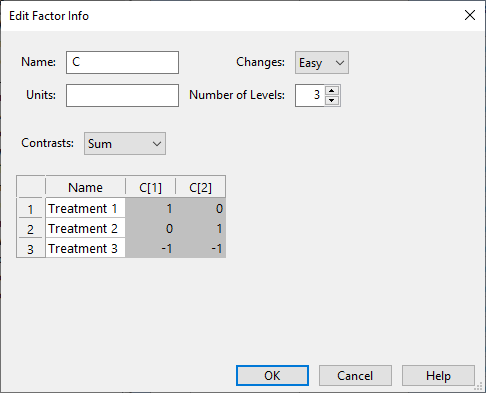# Simulation equations¶

Equations can include references to factor columns, built-in constants, functions, and operations.

Note: A list of all available operators, distribution functions, and mathematical constants can be found here.

## Examples¶

### Example 1: Simple sum of three factors¶

A + B + F


### Example 2: Linear combination of factors¶

2A + B + D/10


### Example 3: Equation involving a ratio of two factors¶

A/F + 3


### Example 4: Equations with exponents, constants, and mathematical functions¶

The equation below involves an expression raised to the power 2, a constant ($$\pi$$), and the square root function,

(A+10)^2 + B*sqrt(_pi^2 + C^2)


### Example 5: Equation which contains a random component¶

2*A + rexp(1)


### Example 6: Equation based on levels of a categorical factor¶

To test for the specific level of a numeric factor, you will need to know the level coding,In this example, C is a 3-level nominal categorical factor. Each of the named levels correspond to a row of two numbers,

• “Treatment 1” above corresponds to C equal to 1 AND C equal to 0,

• “Treatment 2” corresponds to C equal to 0 AND C equal to 1,

• “Treatment 3” corresponds to C equal to -1 AND C equal to -1.

We may test for these conditions using the equality operator ==, the AND operator, &&, and the IF-THEN-ELSE operator, x ? y : z. This last operator may be read as “If x, then y, else z”.

For example, an equation that assigns 5 for “Treatment 1”, 10 for “Treatment 2”, and 20 for “Treatment 3” could be written as follows,

(C==1 && C==0)?5:0 + (C==0 && C==1)?10:0 + (C==-1 && C==-1)?20:0


The first term in this sum tests that C is equal to 1 and C is equal to 0 and assigns a value of 5 if so, otherwise it it assigns 0 so that level doesn’t contribute to the sum. Similar assignments are made for the other two levels.

We could also take advantage of nesting and the fact that there are only 3 levels to write this somewhat more efficiently,

(C==1 && C==0)?5:((C==0 && C==1)?10:20)


Such an equation might represent the contribution of a categorical factor to a cost equation, where each level is associated with a different cost.